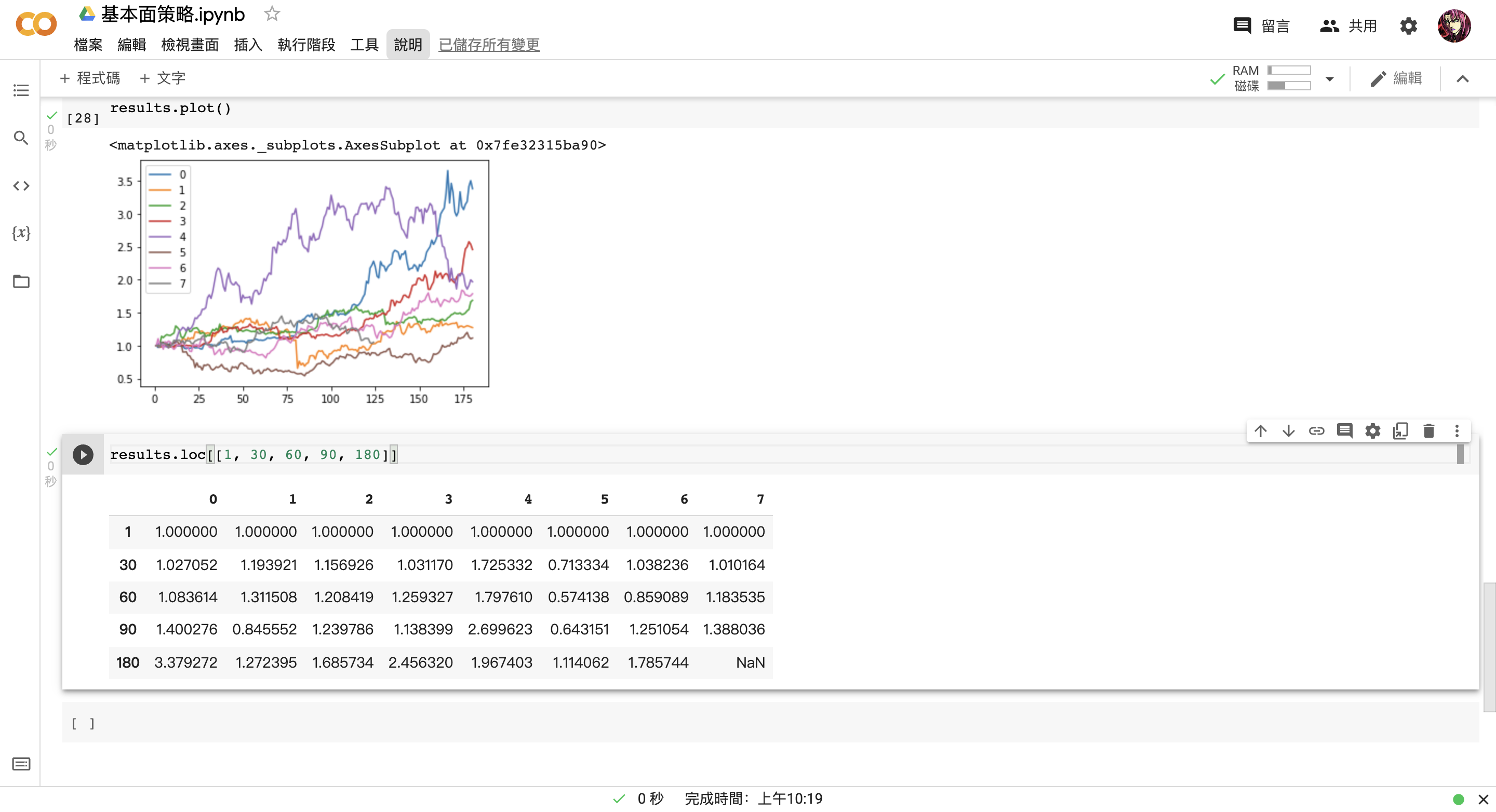# 第三章：打造基本面策略 Hash Rate

### a. 什麼是 Hash Rate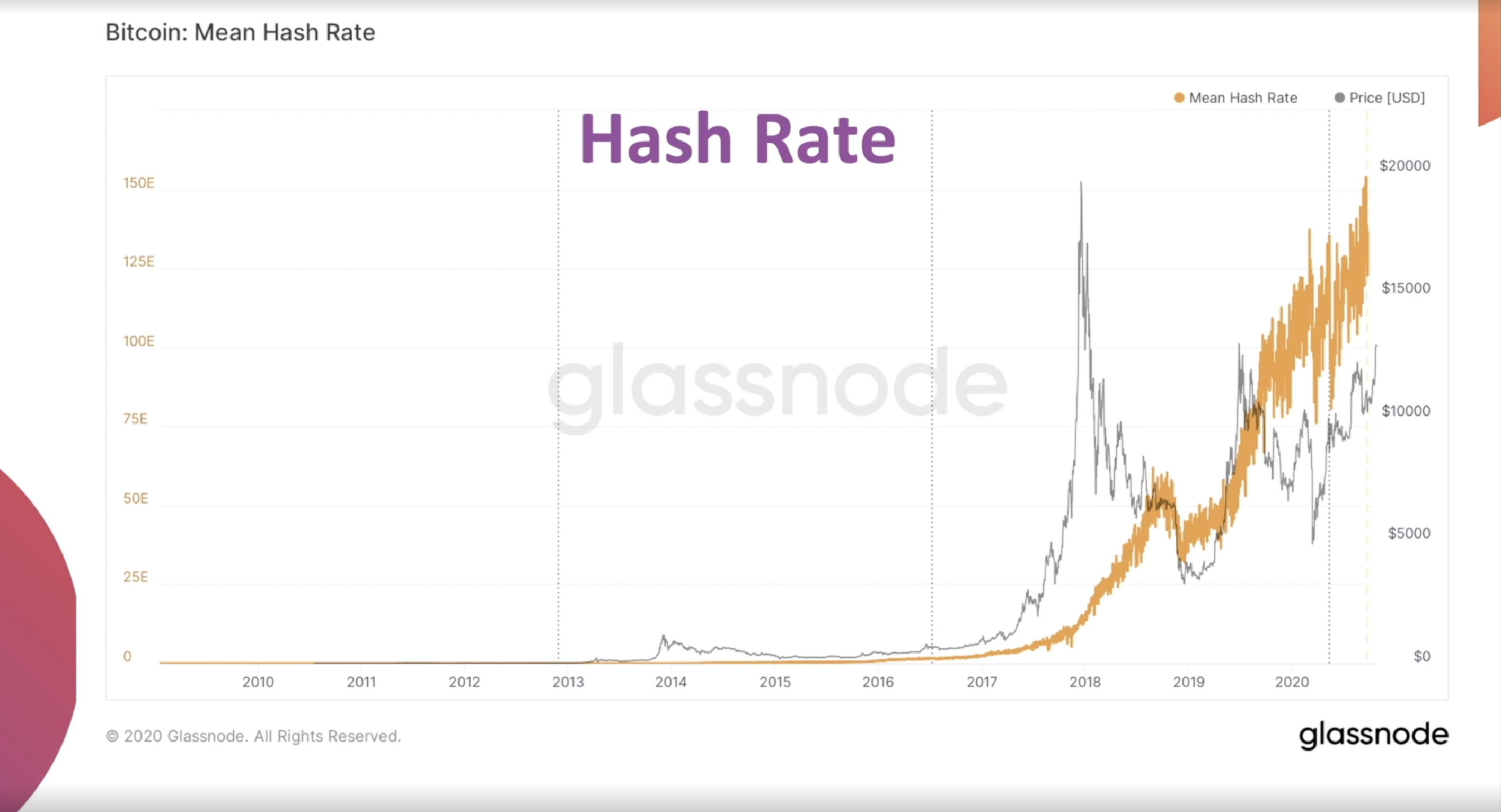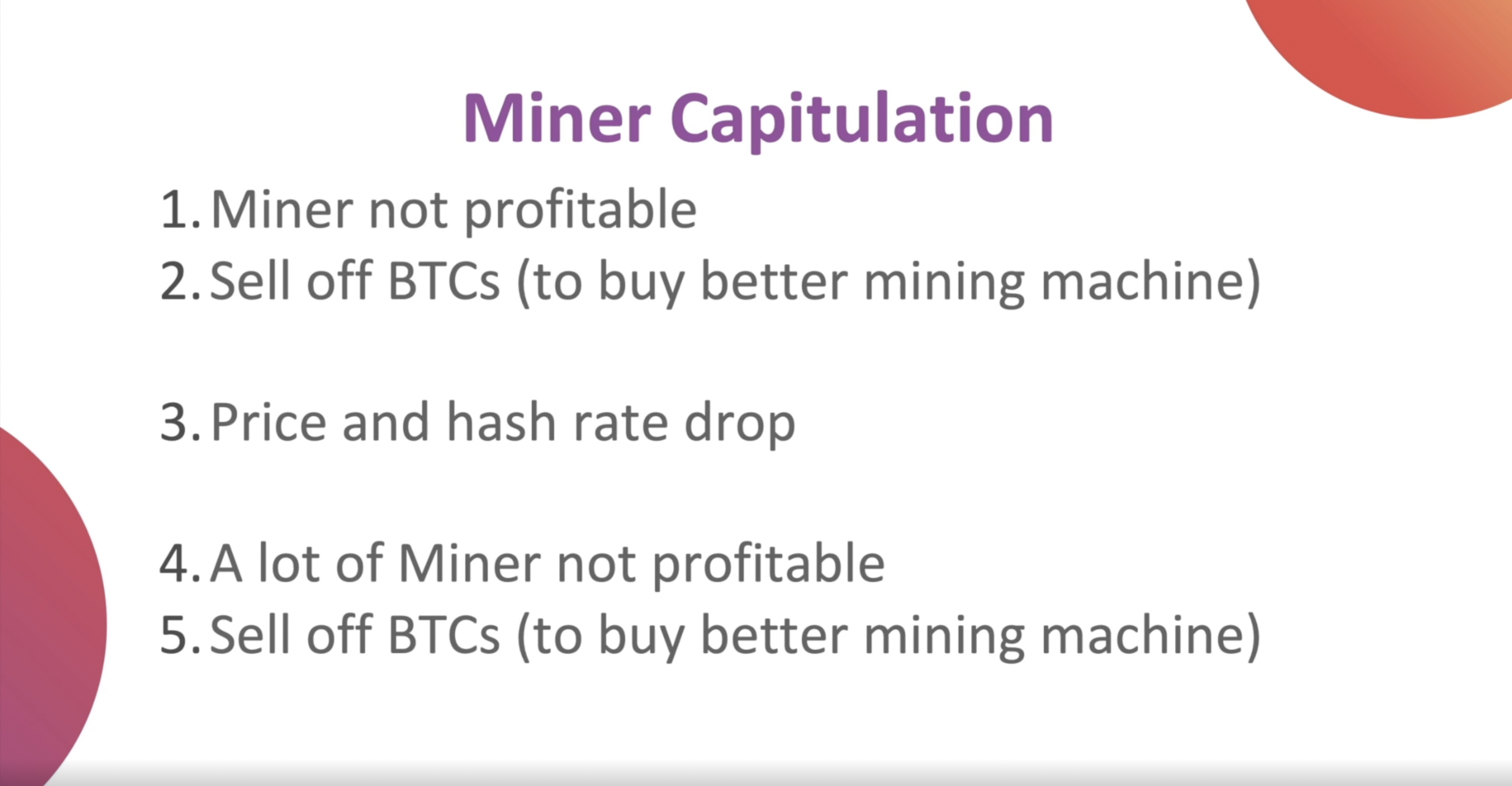### b. 獲取 Hash Rate 資料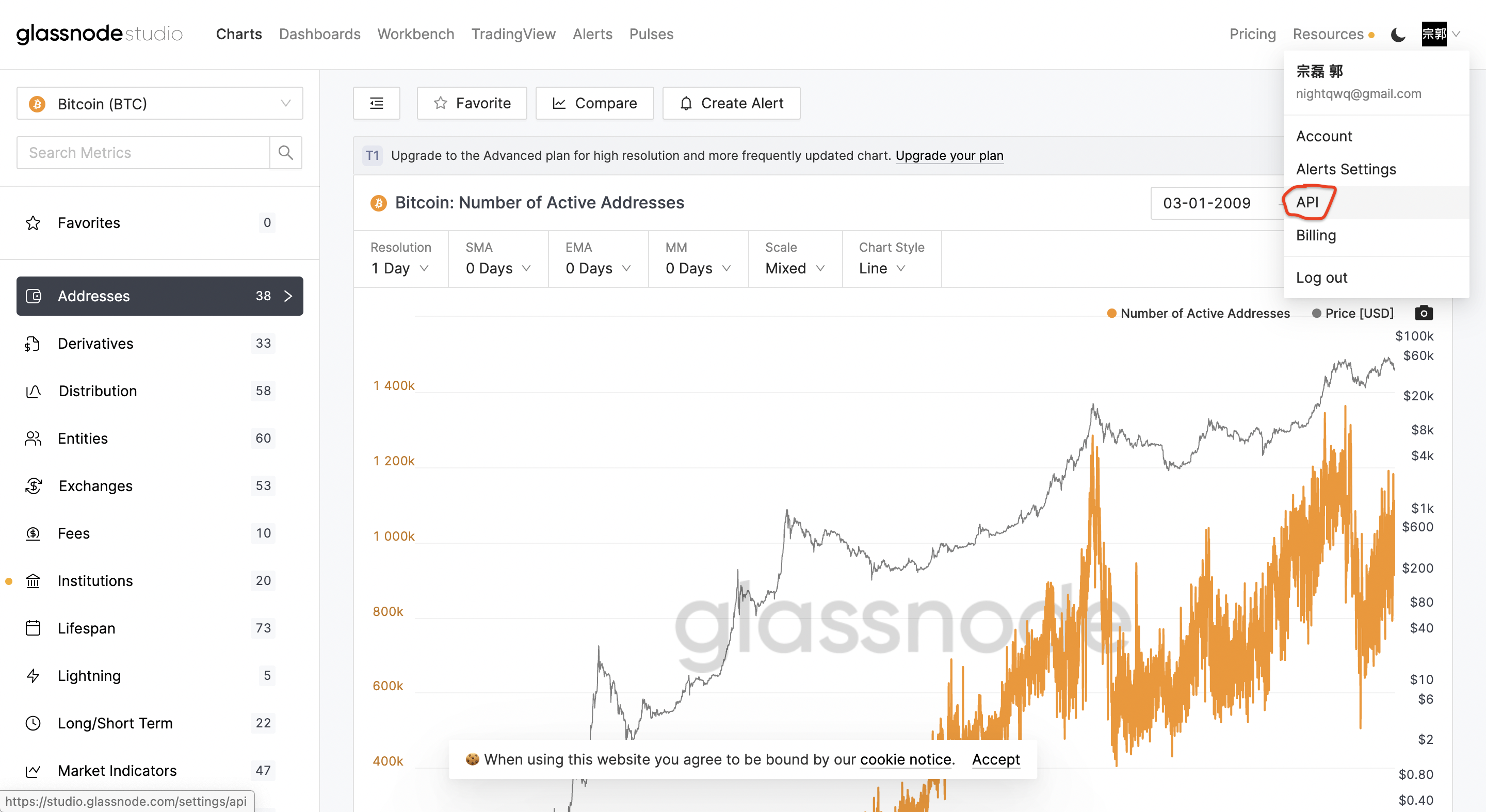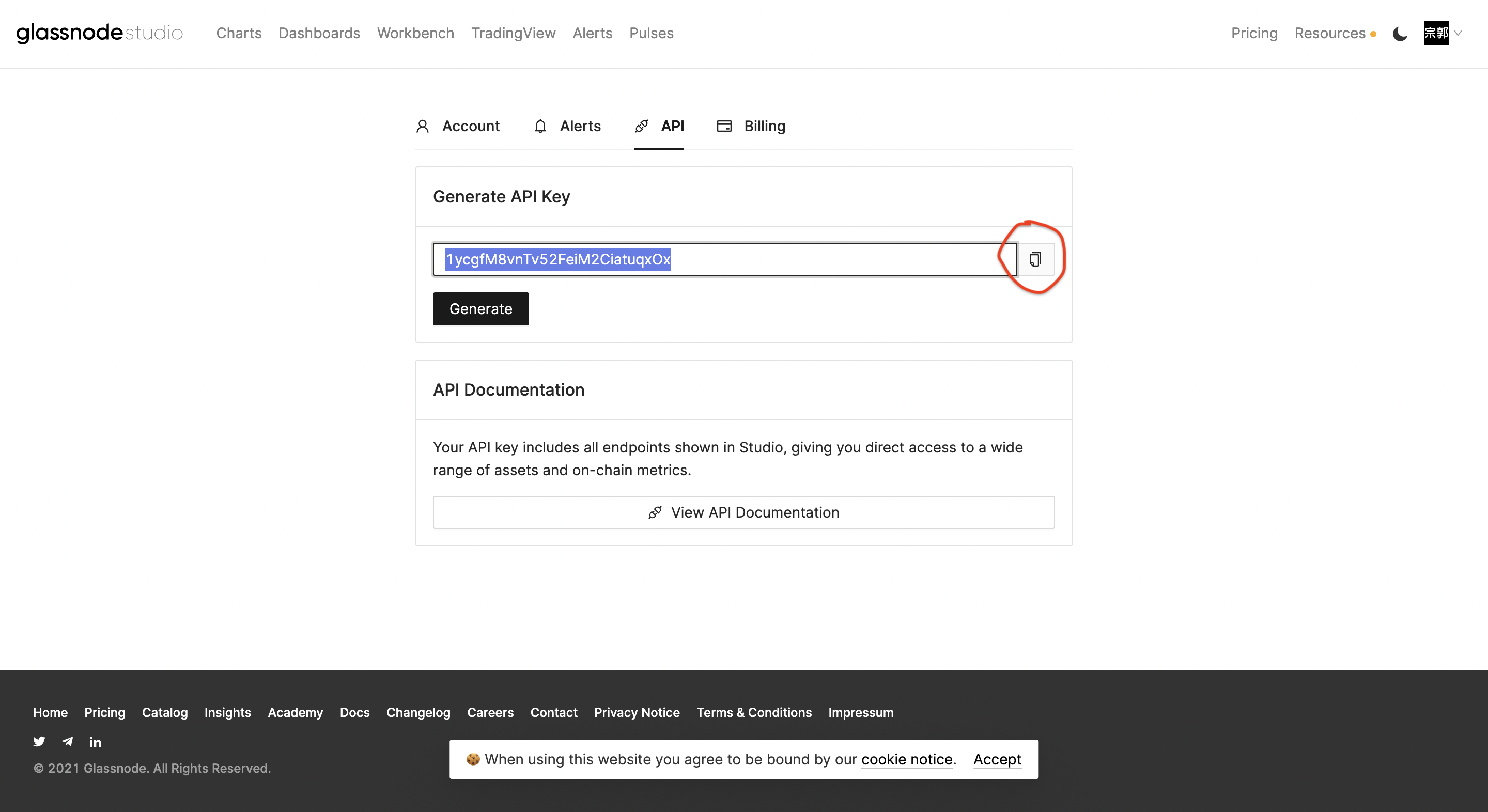### c. Hash Rate 策略撰寫

hash_rate = get_glassnode('你複製下來的網址',
'你複製下來的密鑰', ohlcv.index)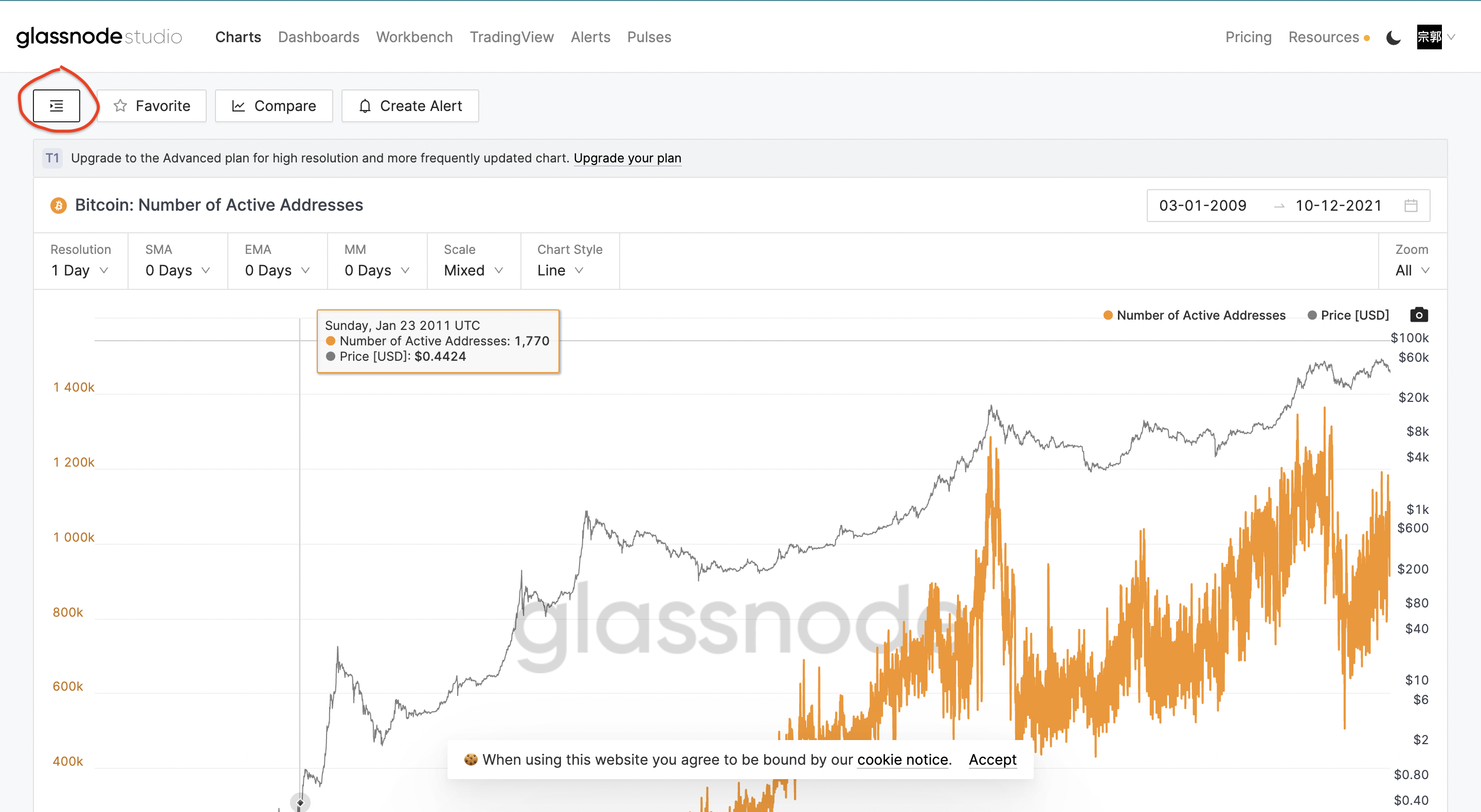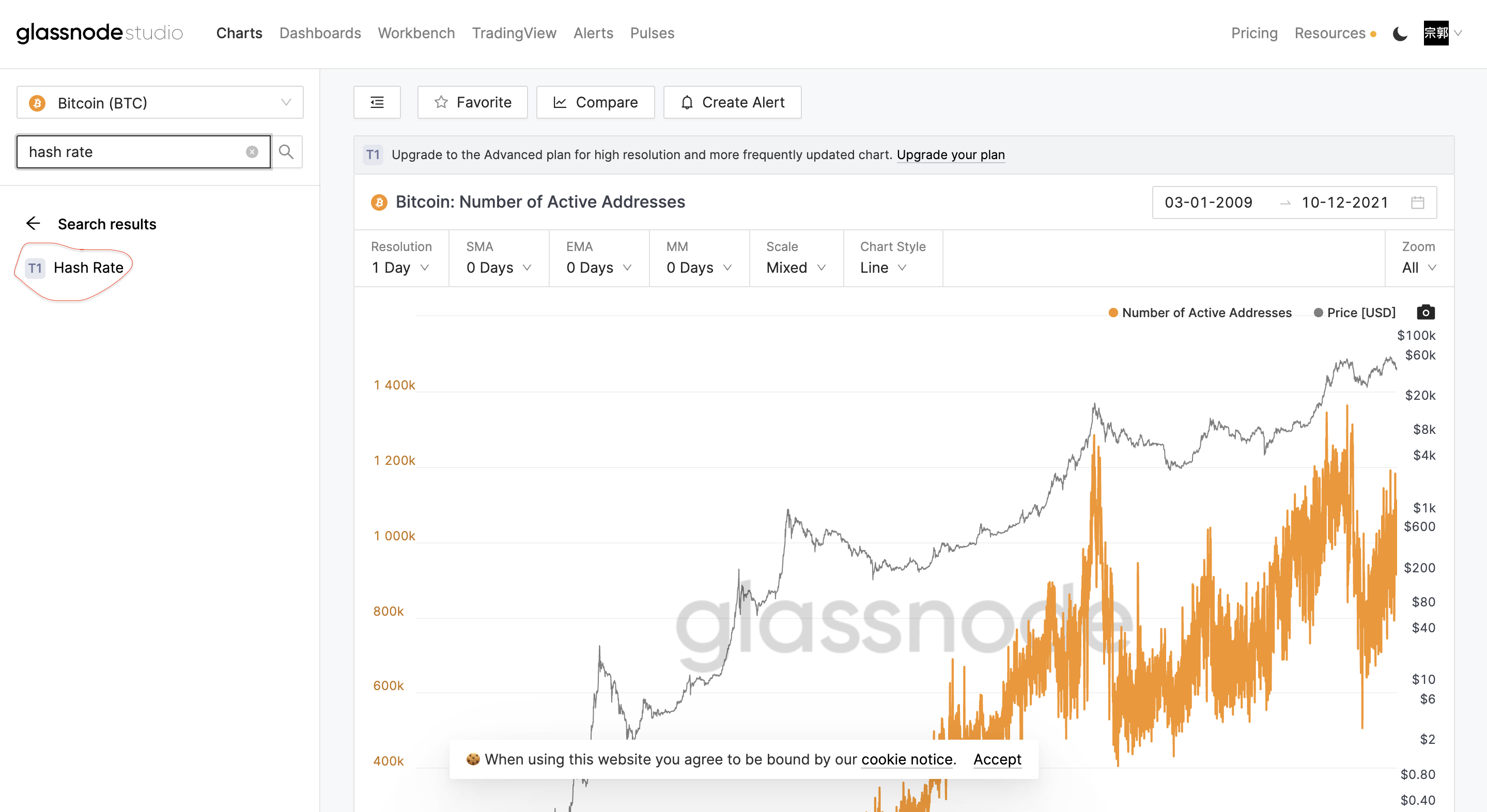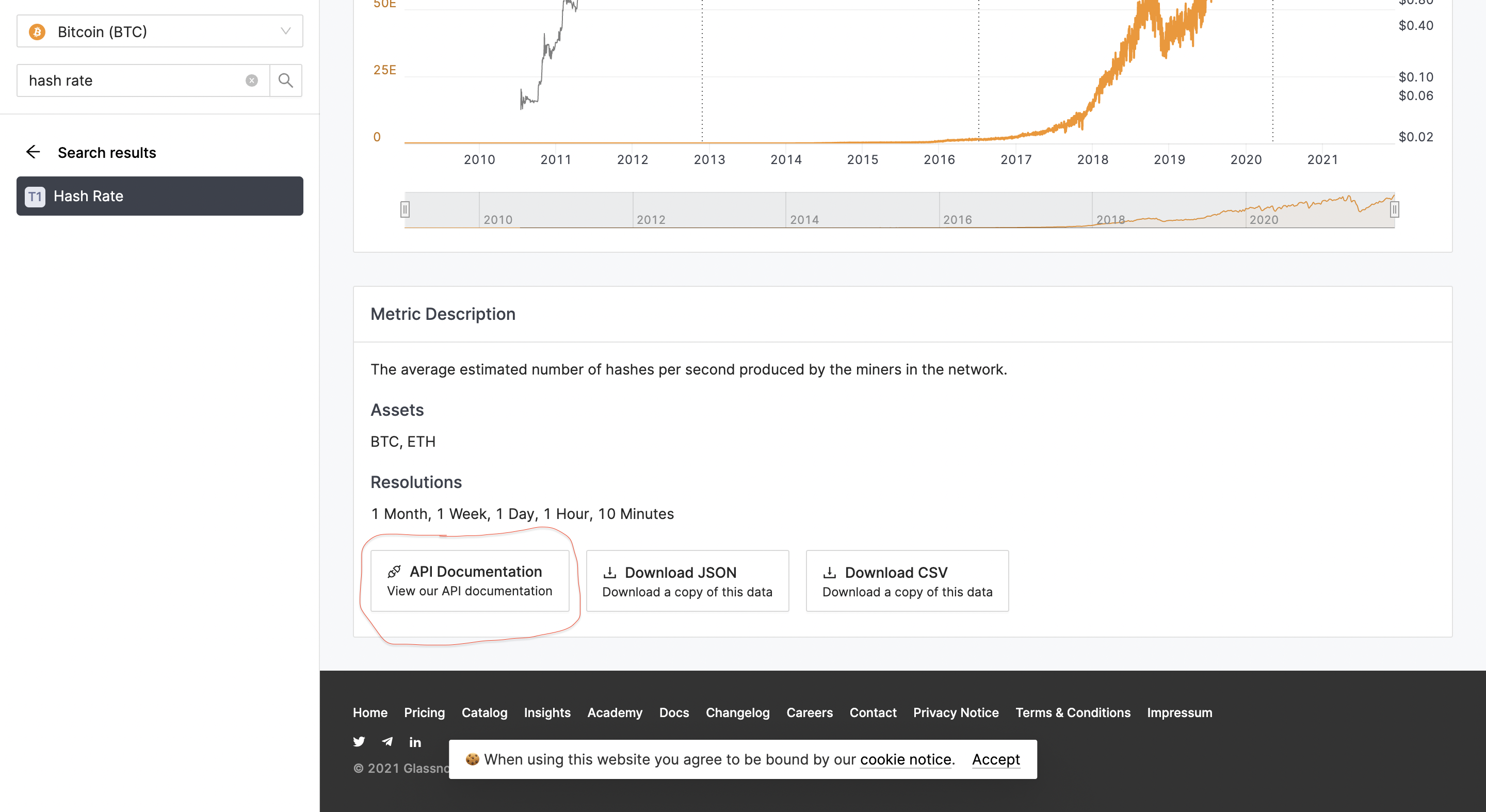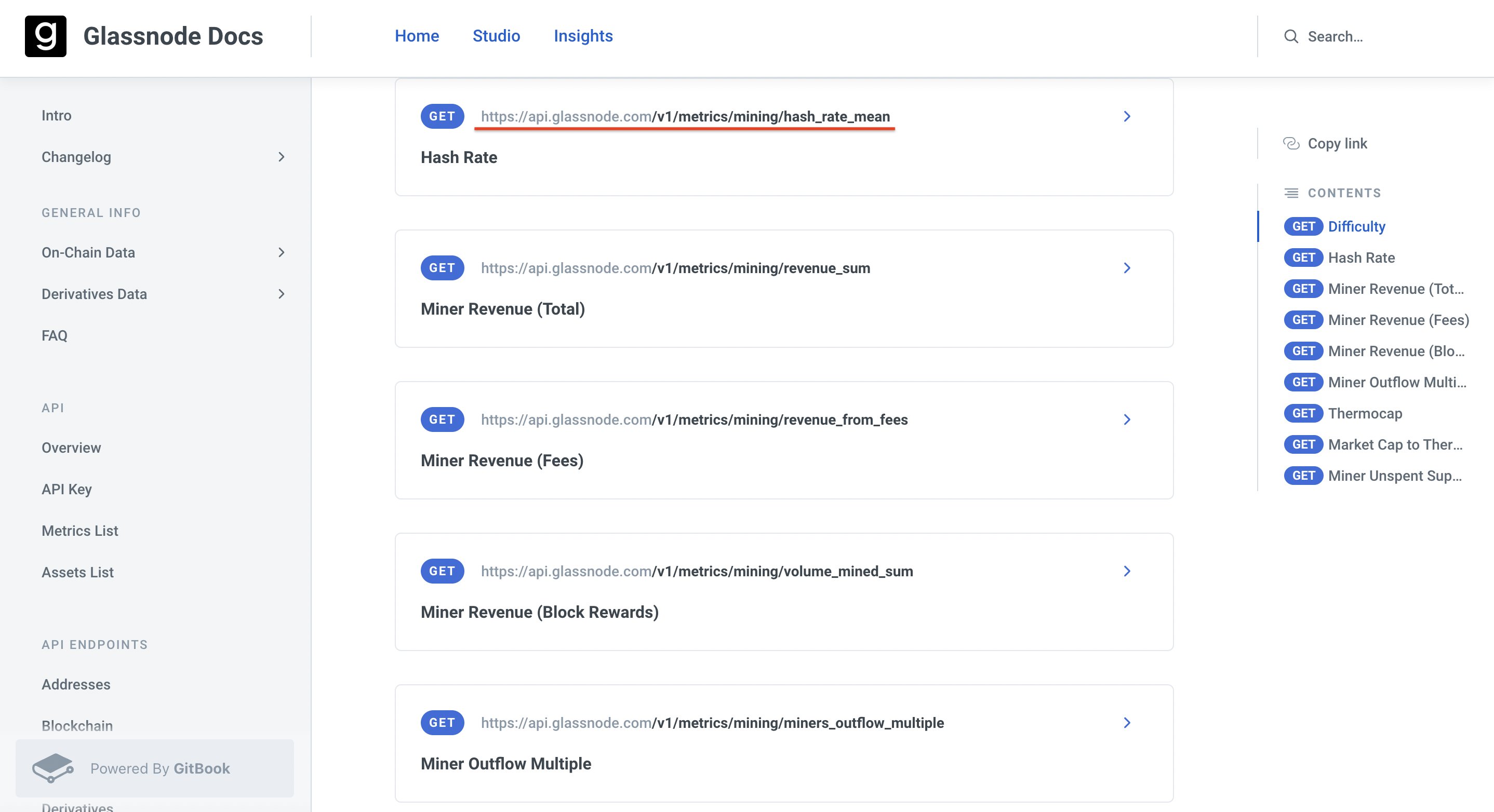hash_rate.plot()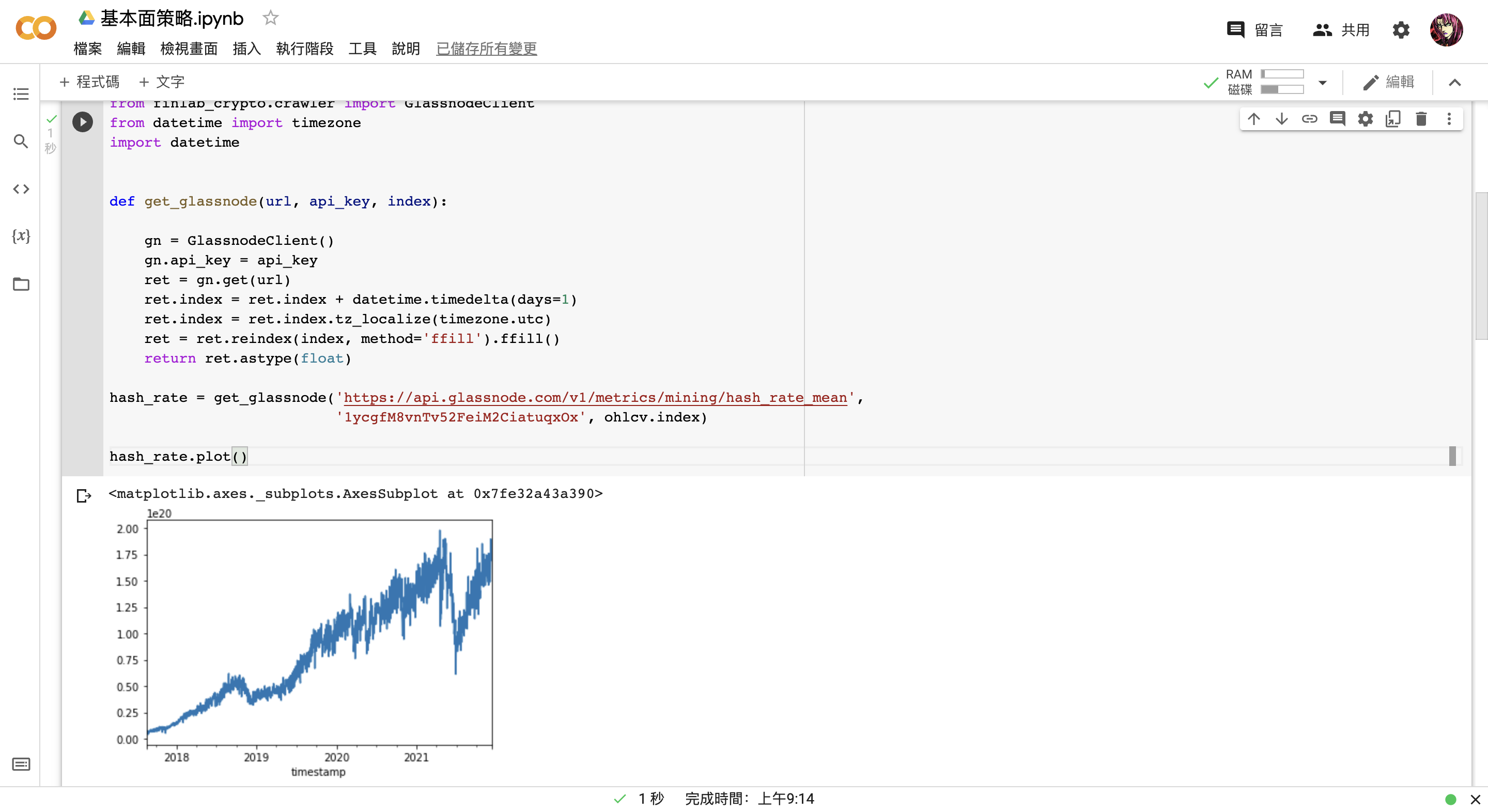hash_rate_ma30 = hash_rate.rolling(30).mean()

hash_rate_ma60 = hash_rate.rolling(60).mean()

hash_rate_ma30.plot()

hash_rate_ma60.plot()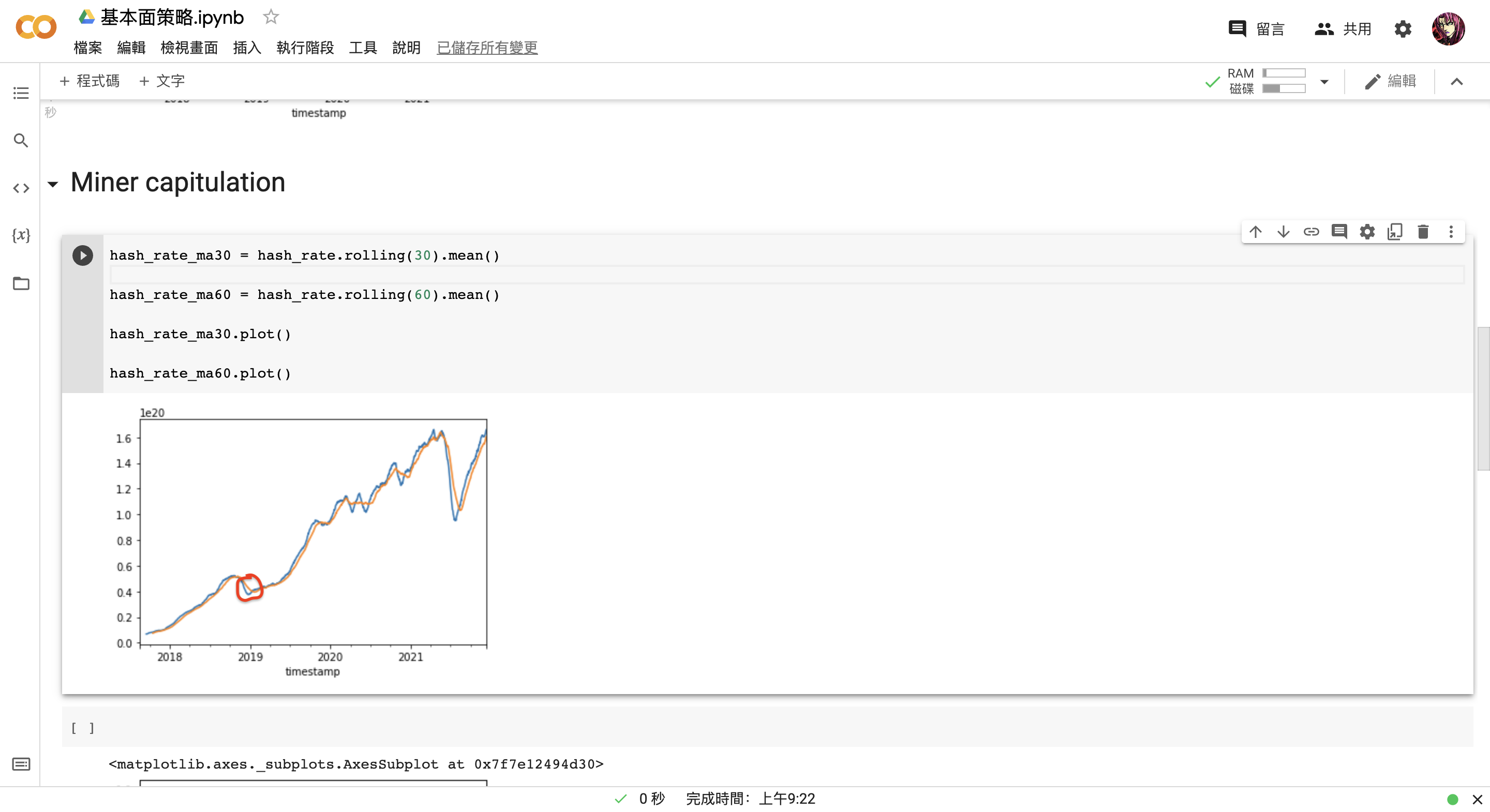cross = (hash_rate_ma30 > hash_rate_ma60) & (hash_rate_ma30.shift() < hash_rate_ma60.shift())

cross.astype(int).plot()


ohlcv.close.plot(secondary_y = True)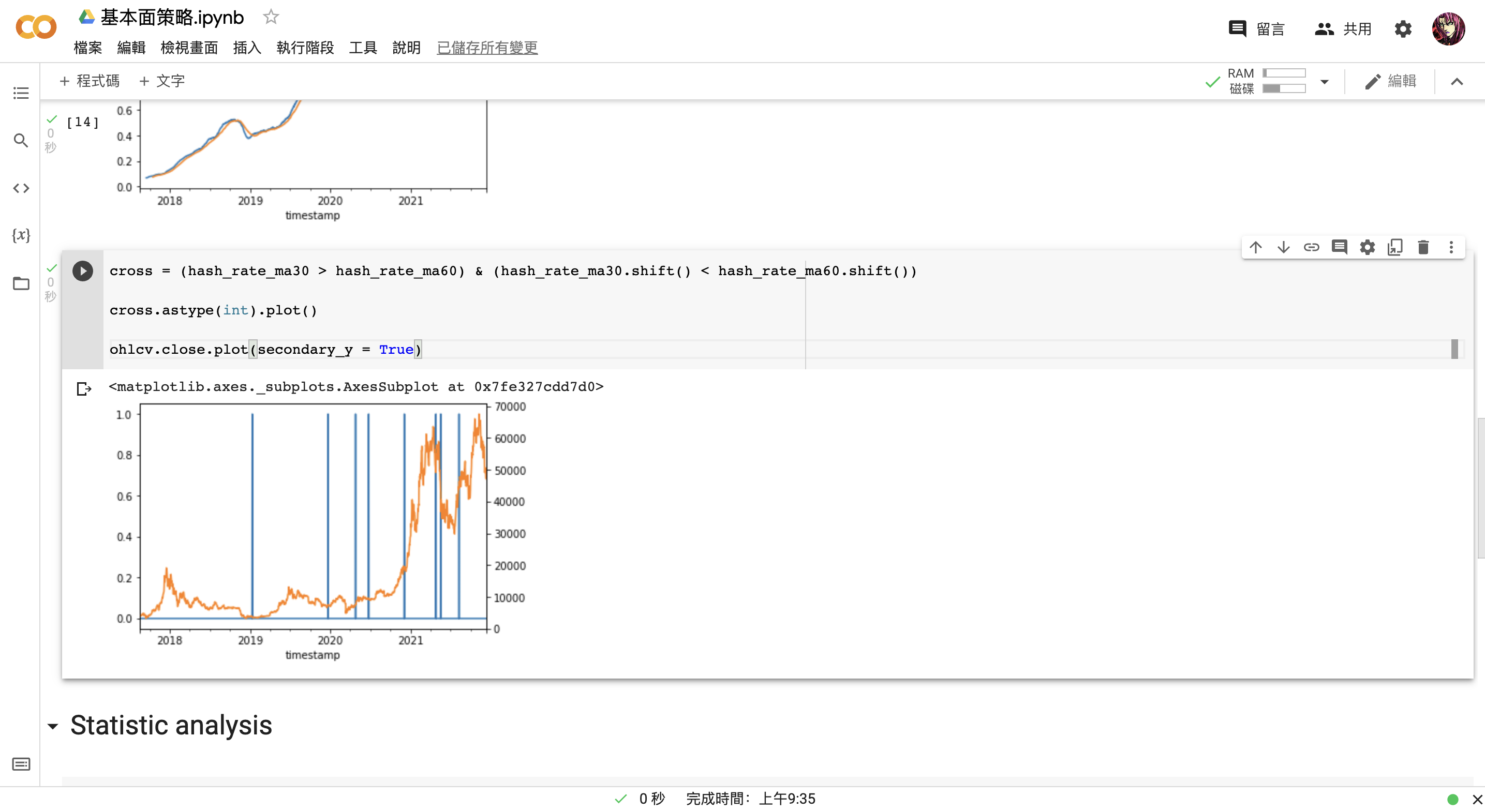for d in range(1, 181):

dprice = ohlcv.close[cross.shift(d).fillna(False)].values

results[d] = pd.Series(dprice)

results = pd.DataFrame(results)

results = results.transpose()

results


（倒數第二行程式碼能夠讓表格旋轉九十度）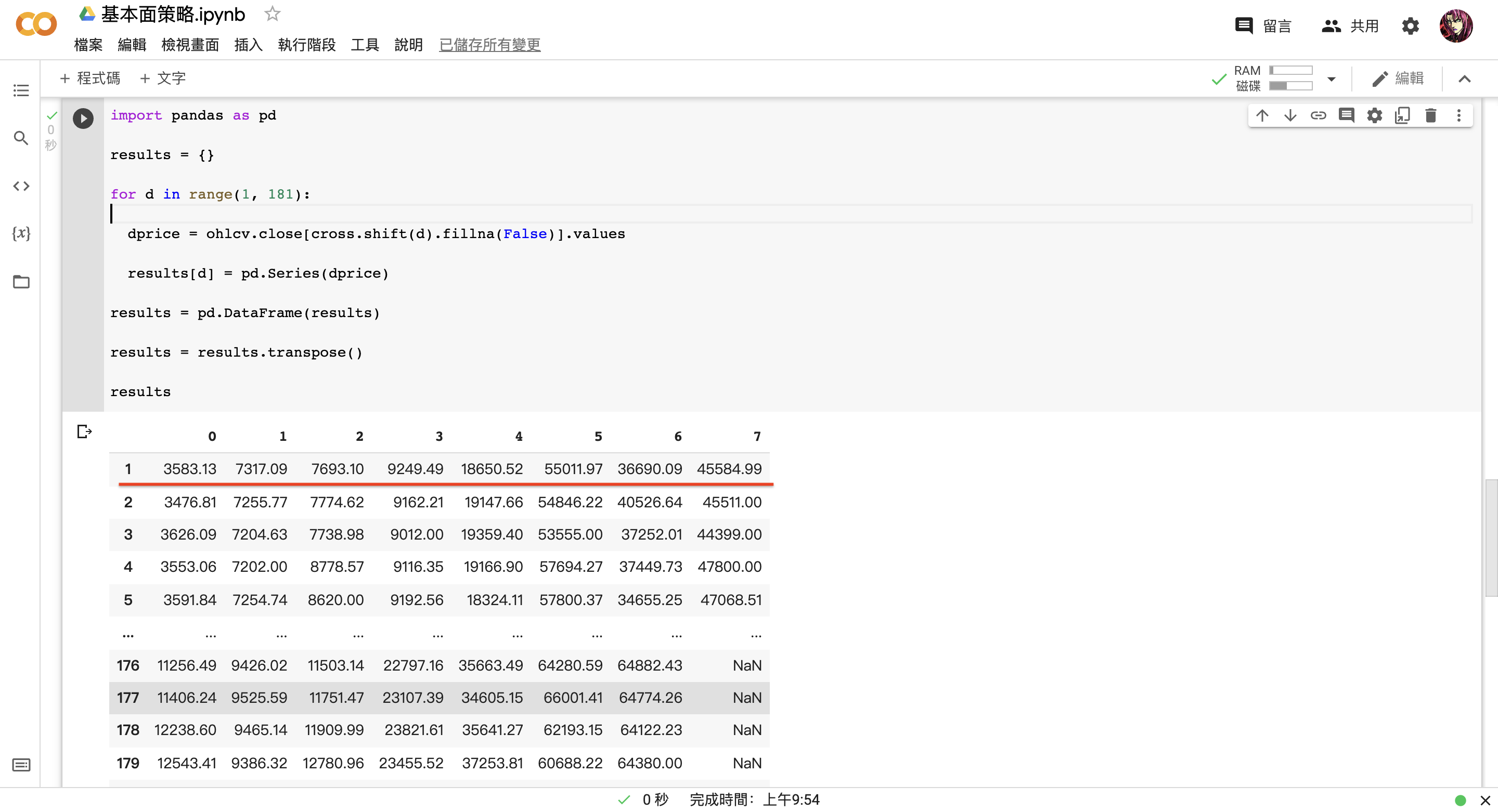results = results.div(results.iloc)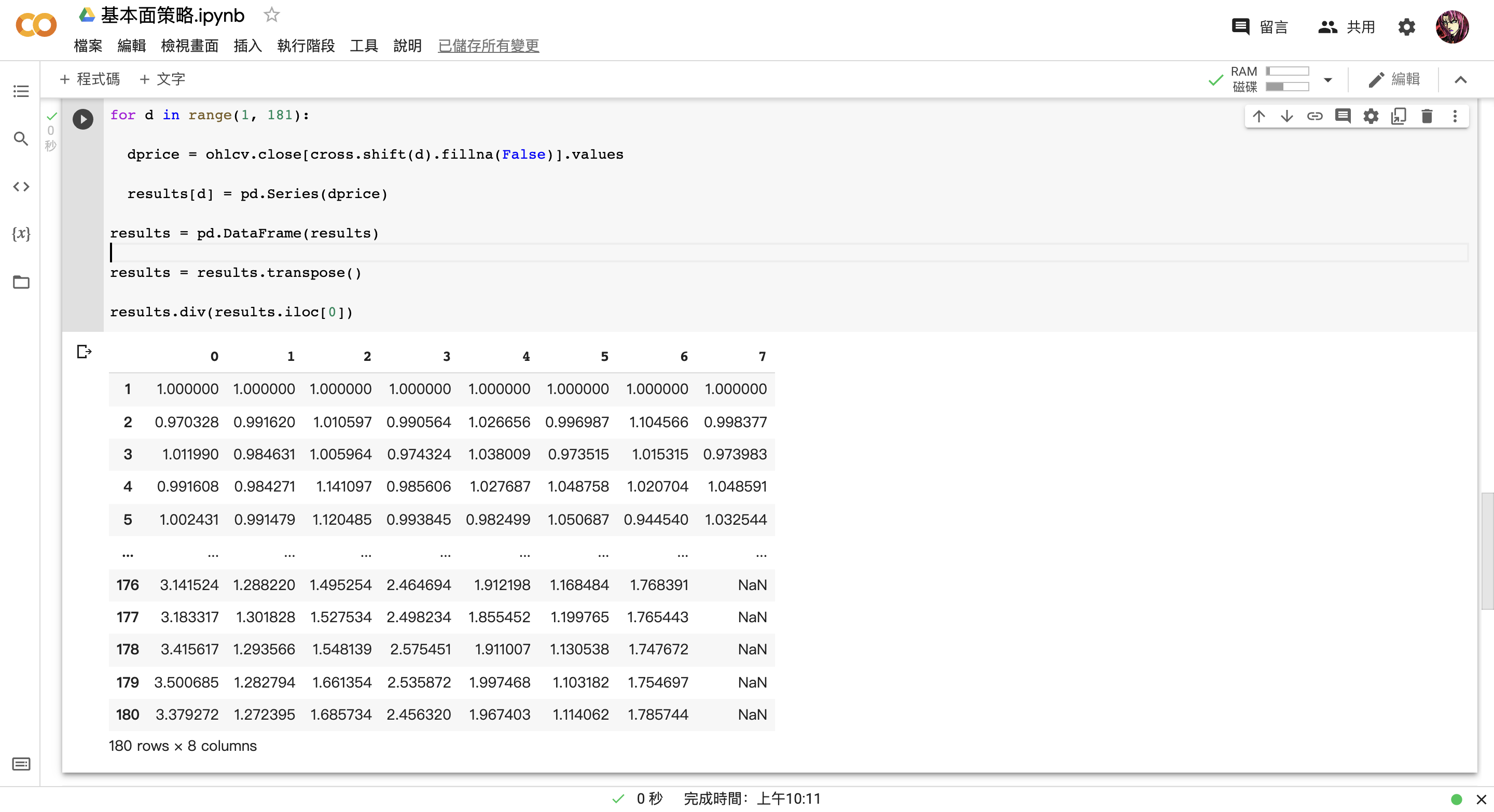results.plot()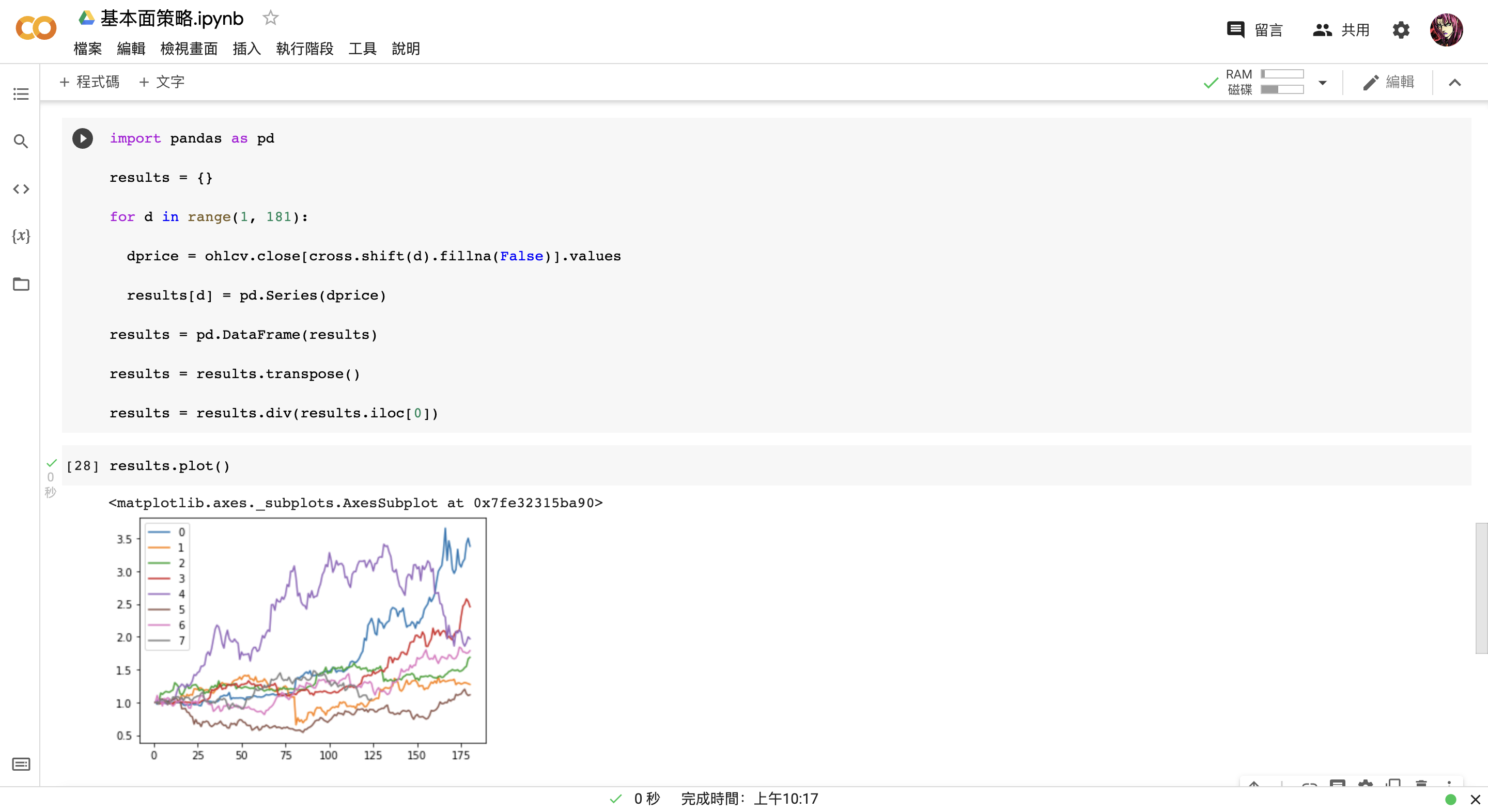results.loc[[1, 30, 60, 90, 180]]